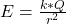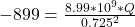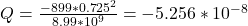## The electric field everywhere on the surface of a thin, spherical shell of radius 0.725 m is of magnitude 899 N/C and points radially toward

Question

The electric field everywhere on the surface of a thin, spherical shell of radius 0.725 m is of magnitude 899 N/C and points radially toward the center of the sphere.What is the net charge within the sphere’s surface

in progress 0
2 weeks 2021-09-05T16:46:22+00:00 1 Answers 0 views 0

Q = -52.56 nC

Explanation:

The electric field outside a spherical shell is given bywhere:

Q is the net  charge

r is the radius of the spherical shell and E is the electric field magnitude.

Given:

r = 0.725 m

since the field points radially toward the center of the sphere, E = -899 N/C.

k is a constant with a value = 8.99 x 109 Nm²/C².Q = -52.56 nC